### Home > CC4 > Chapter 7 > Lesson 7.1.3 > Problem7-39

7-39.
1. Rewrite each of the following expressions in a simpler form. Homework Help ✎

2.  a.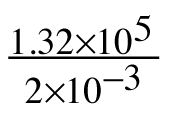b.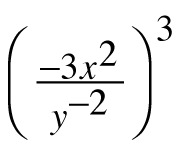c. (3x + 4)(4y – 1) d. (x – 2)2 + 2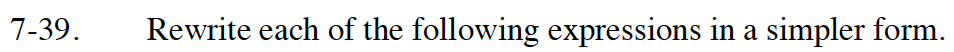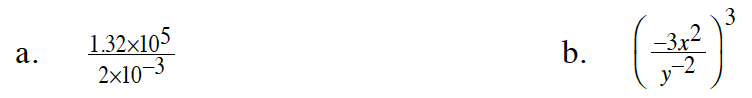Read Math Notes box in Lesson 1.3.1 on scientific notation.

6.6 × 107

Read Math Notes box in Lesson 1.3.2 to review the rules of exponents.

Multiply the all of the exponents in the parentheses by 3. Remember 3 = 31. In the numerator you have
( -31 · 3 · x2 · 3). In the denominator you will have y-2 · 3.

Make sure final answer has no negative exponents.

-27x6y6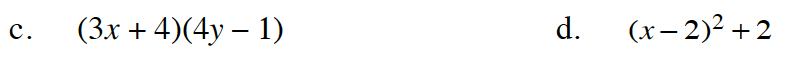Use a generic rectangle.
Read Math Notes box in Lesson 3.2.5 for more help.

Remember (x − 2)² is equivalent to (x − 2)(x − 2).
See part (c) for more help.